Successfully reported this slideshow.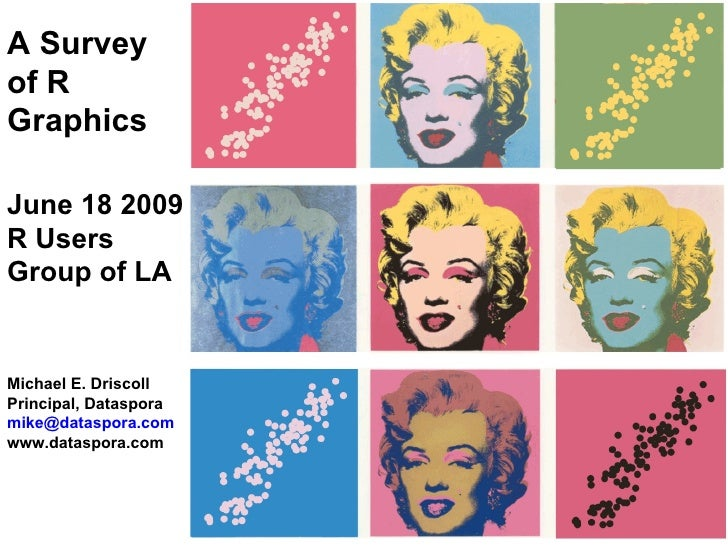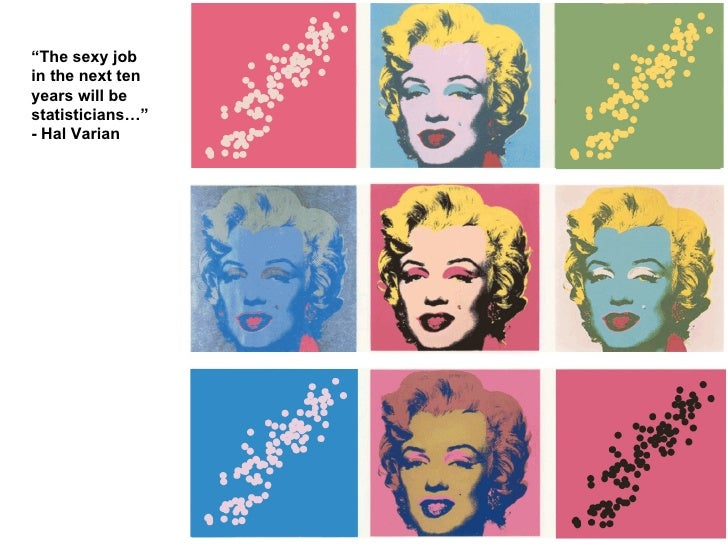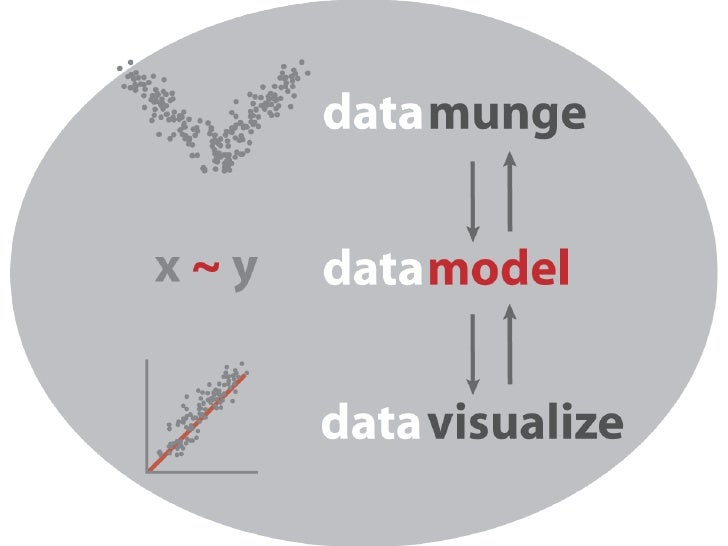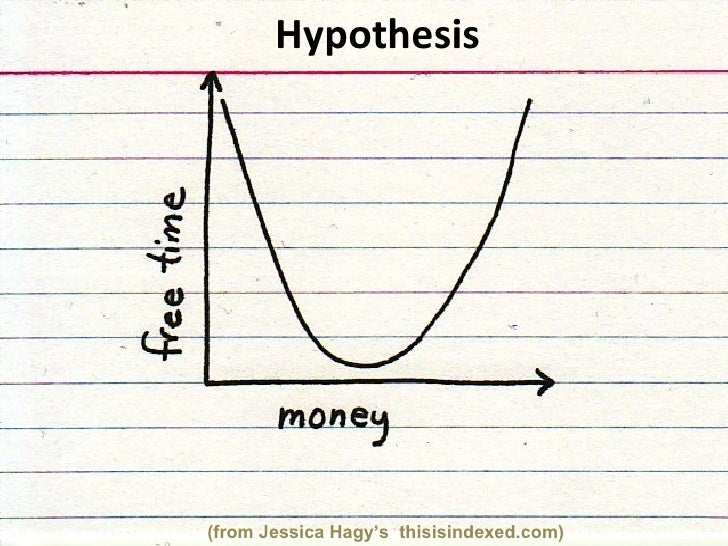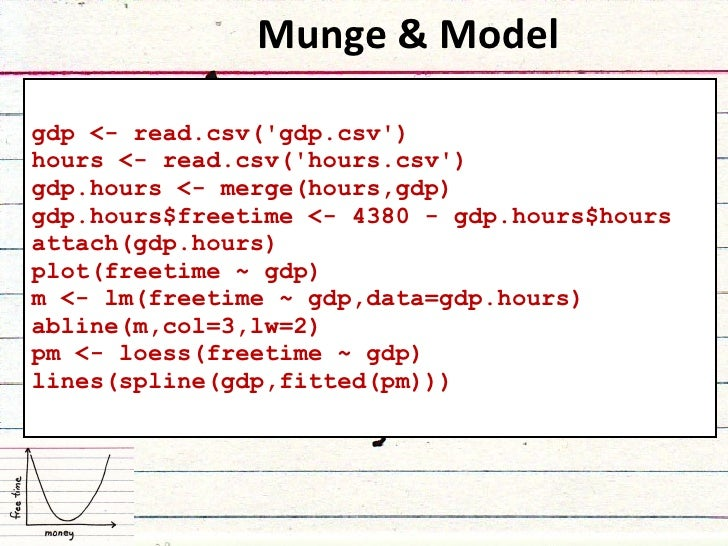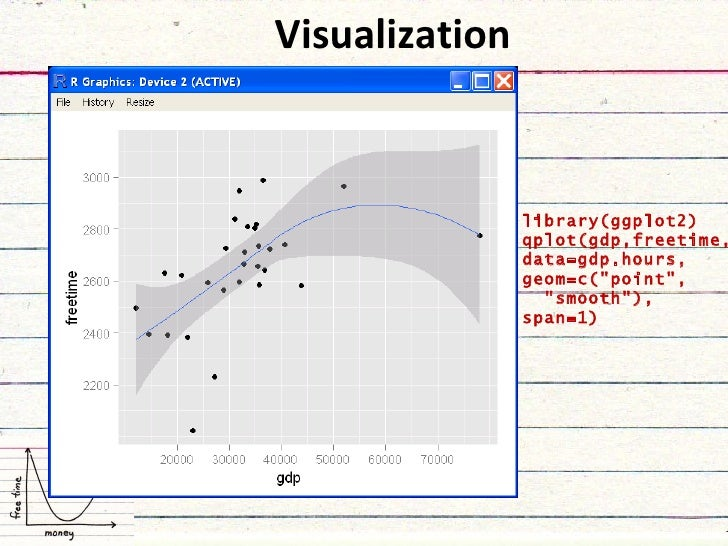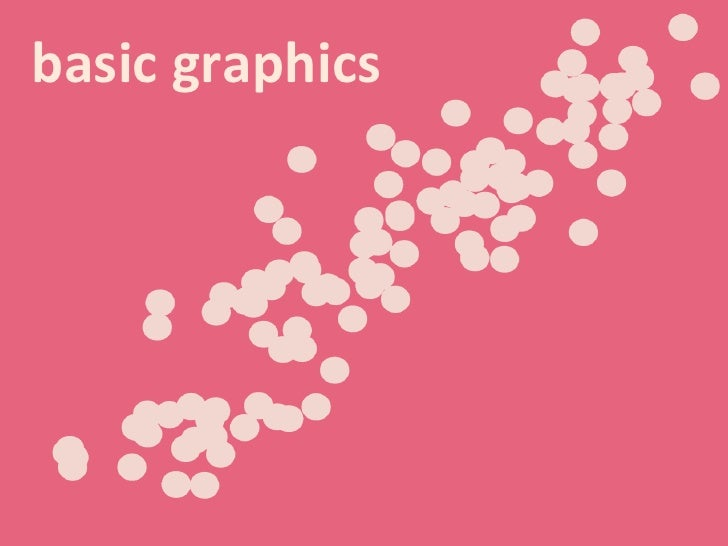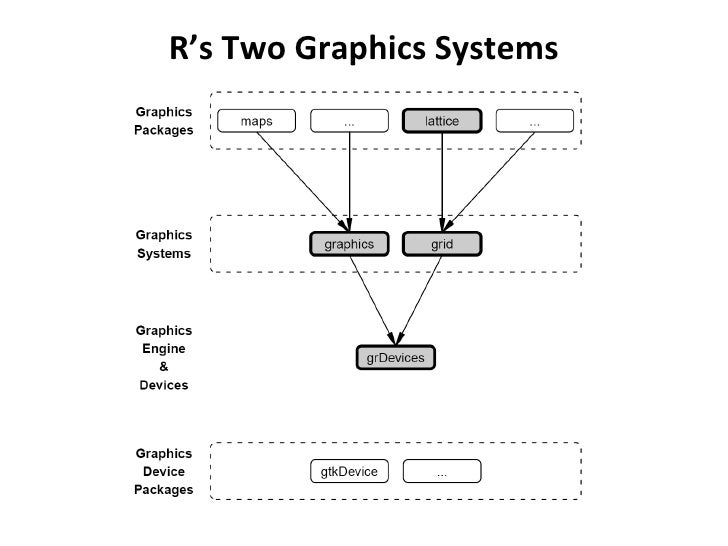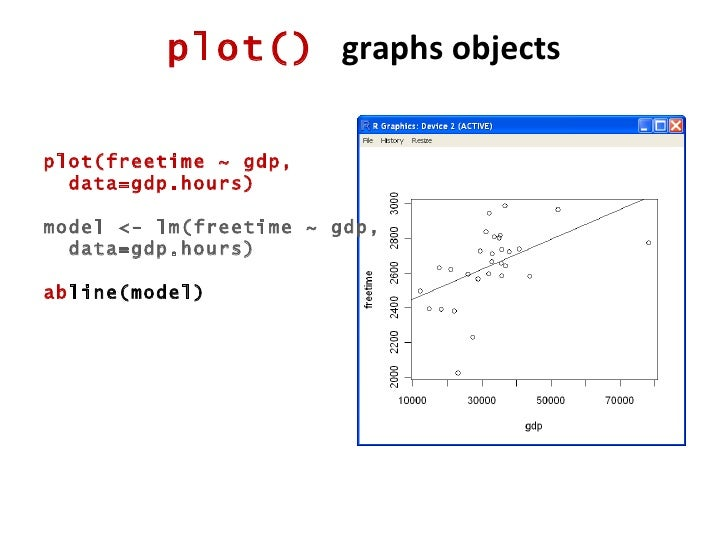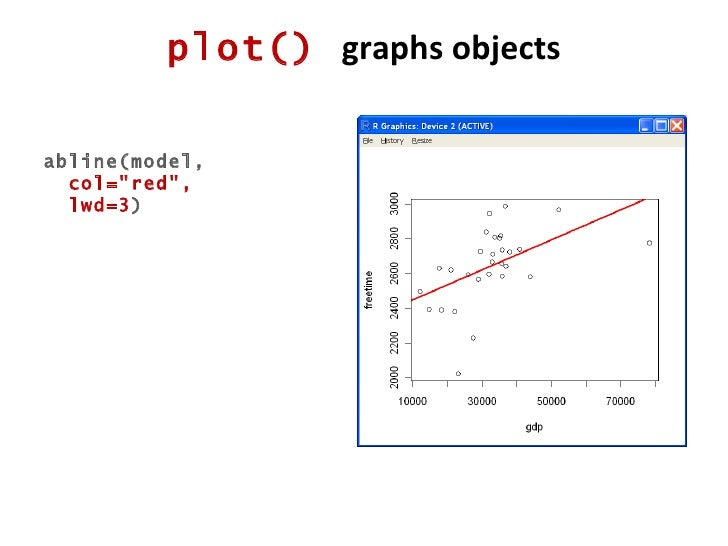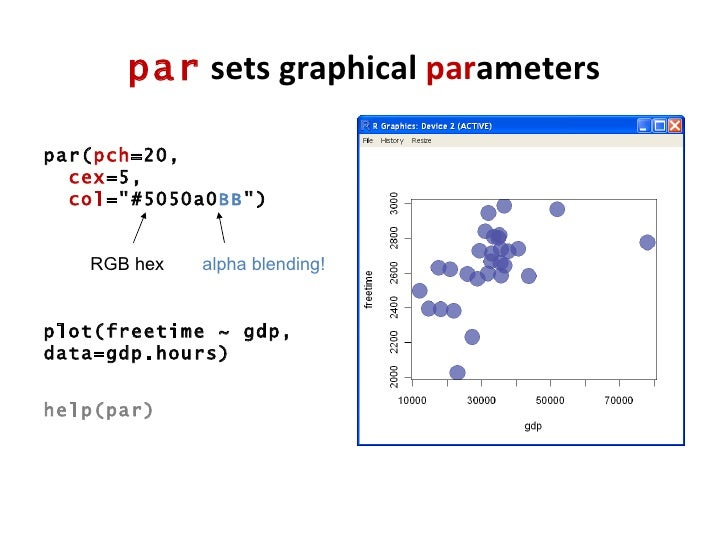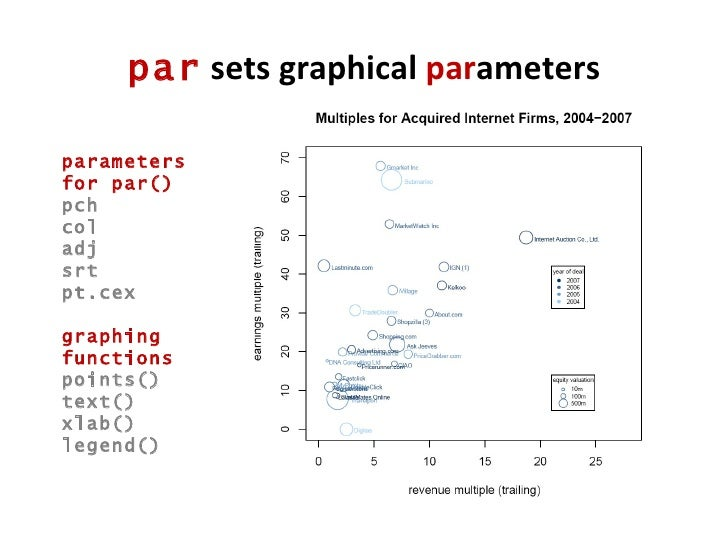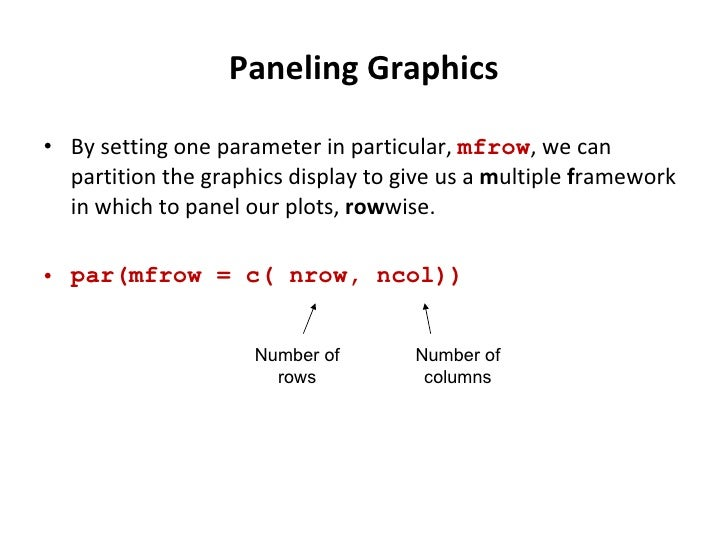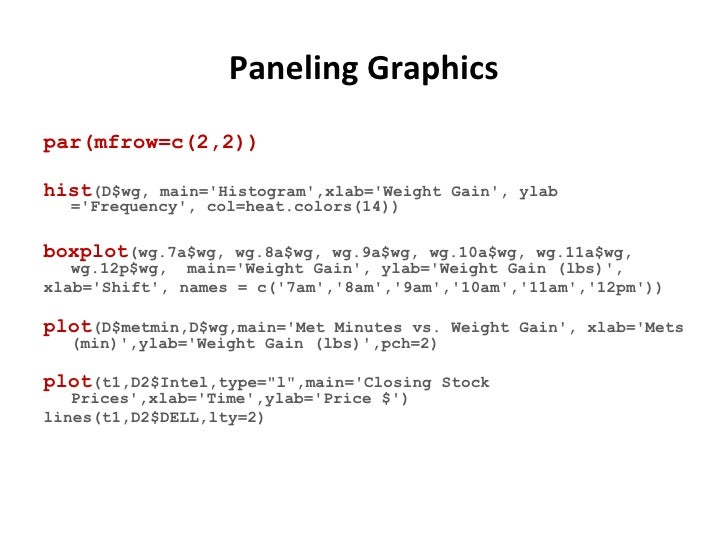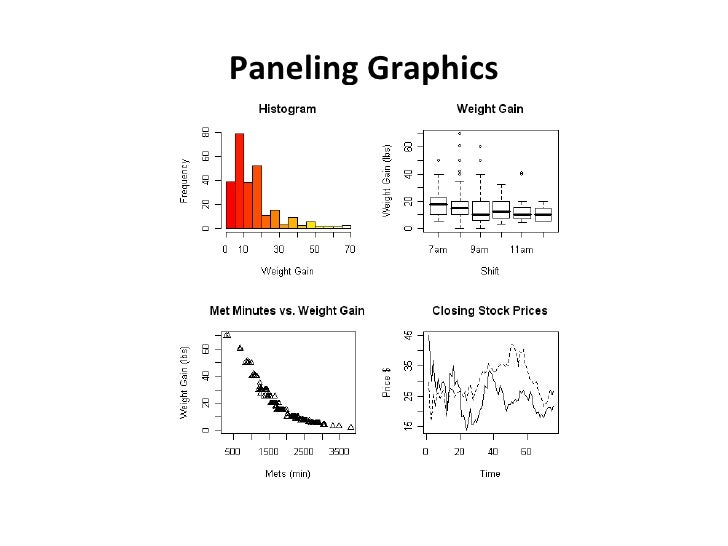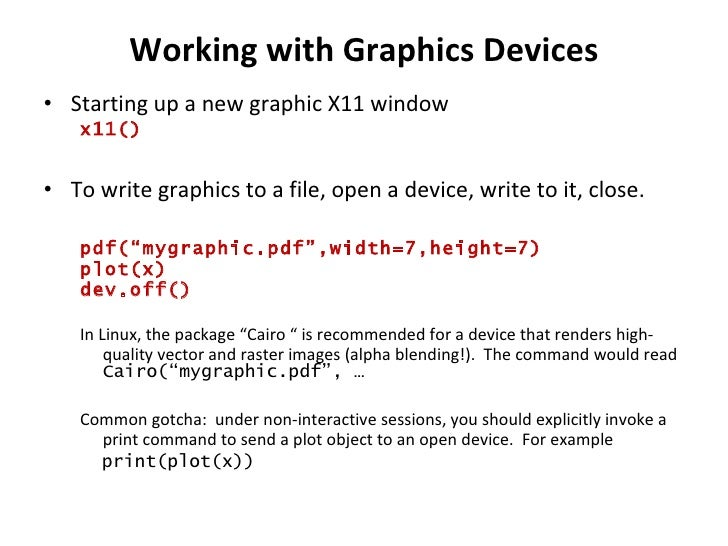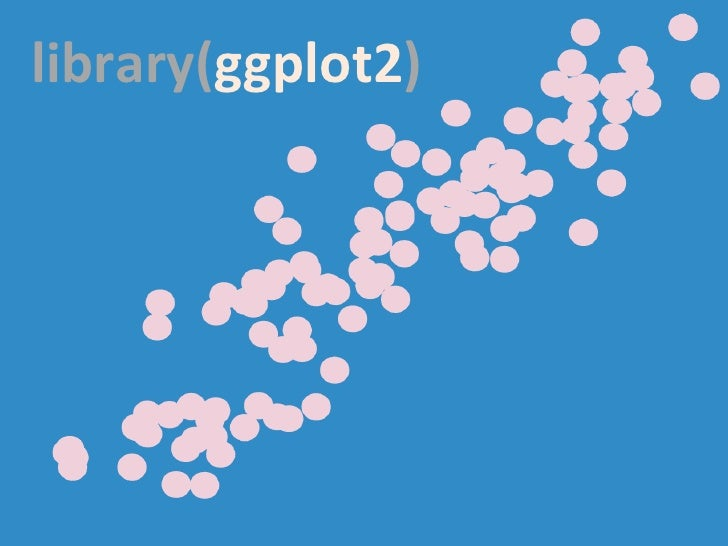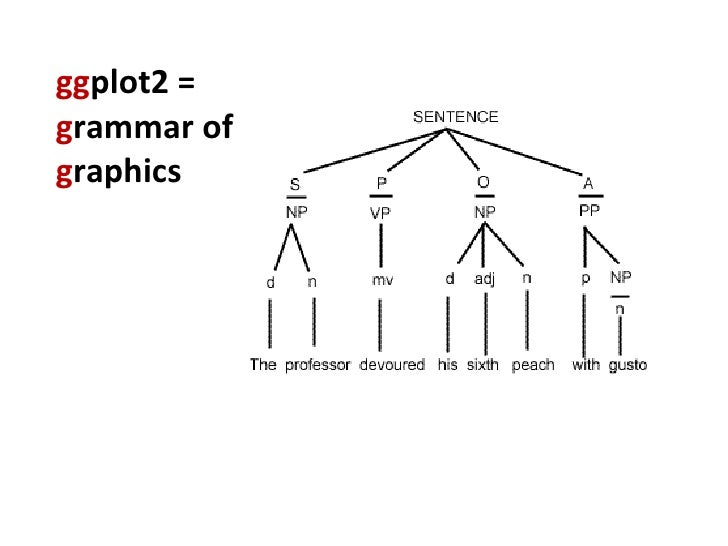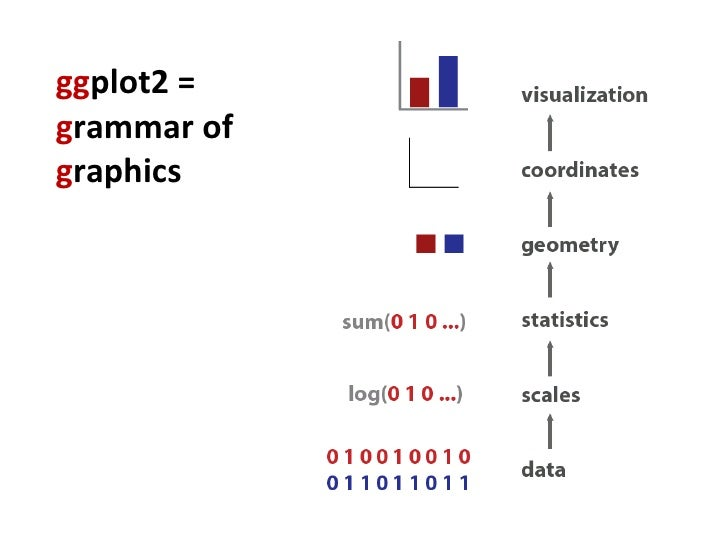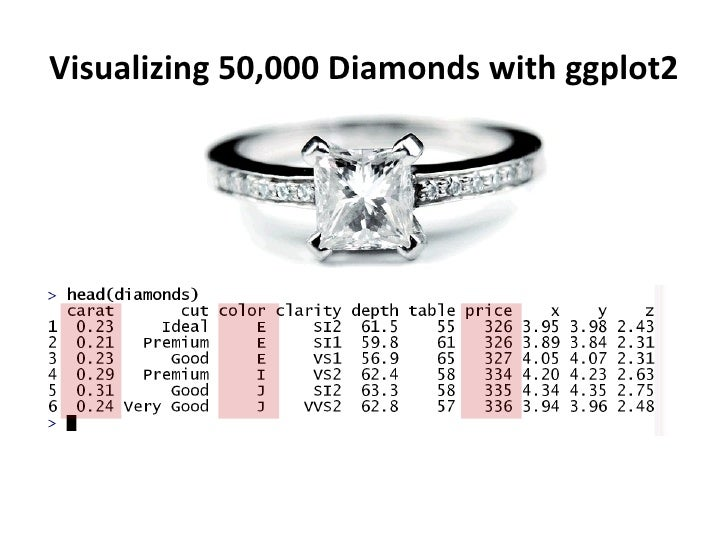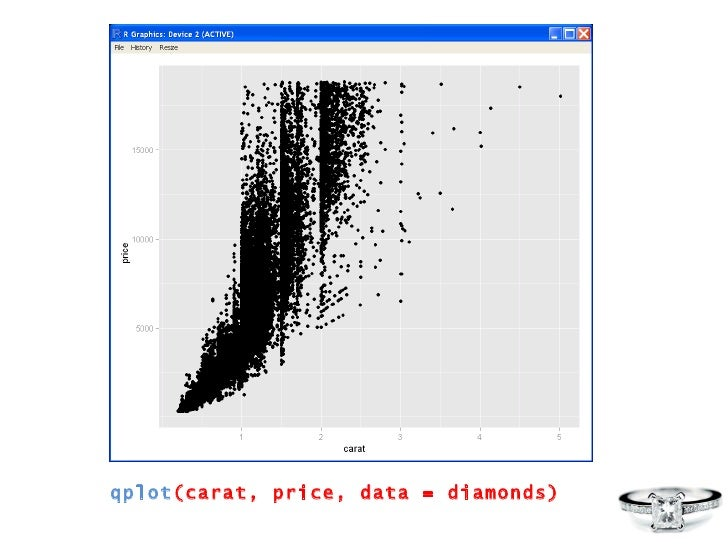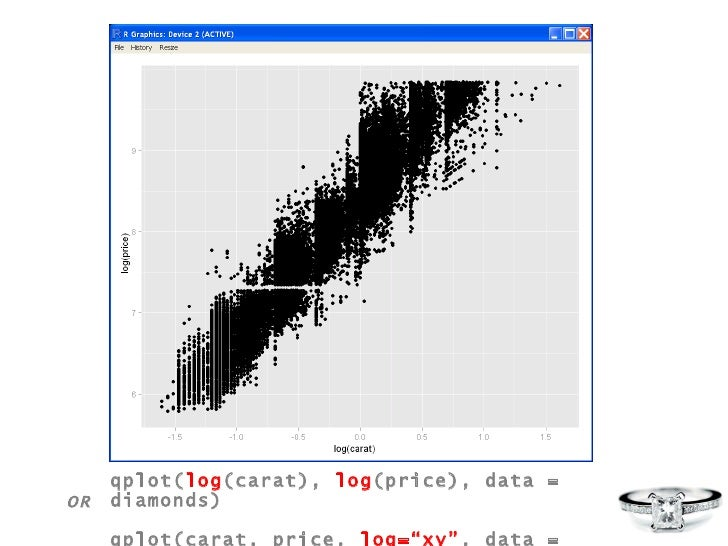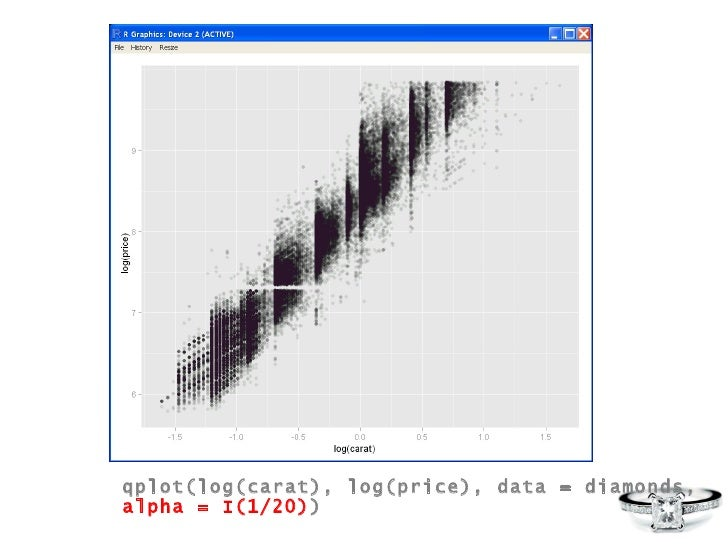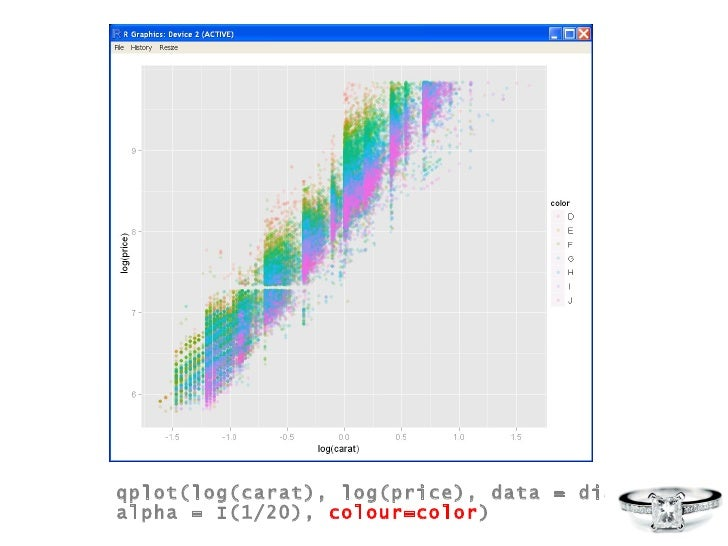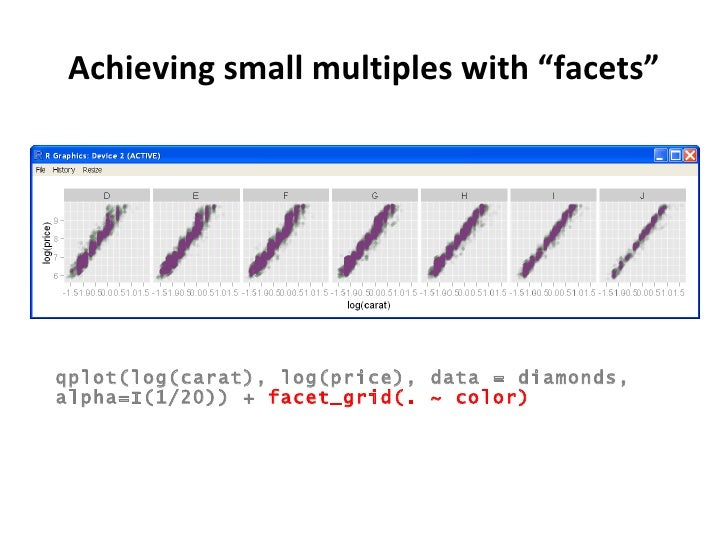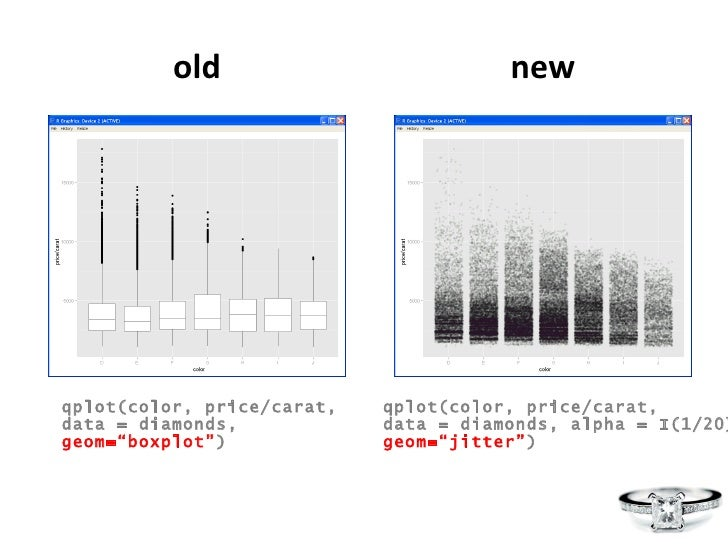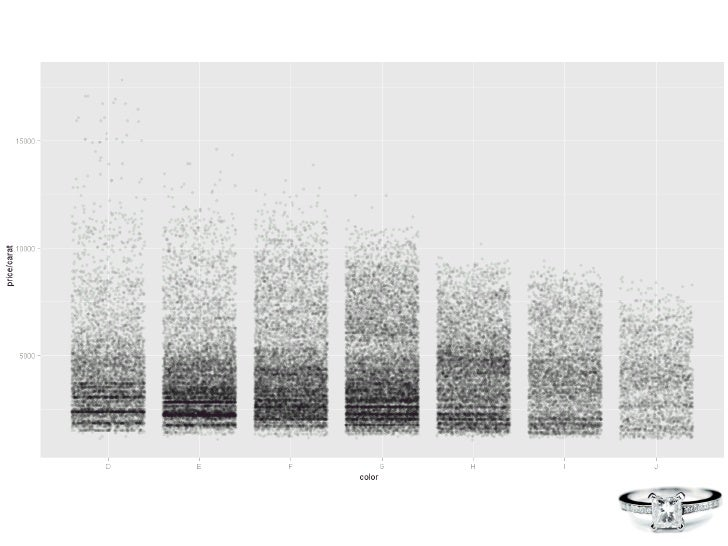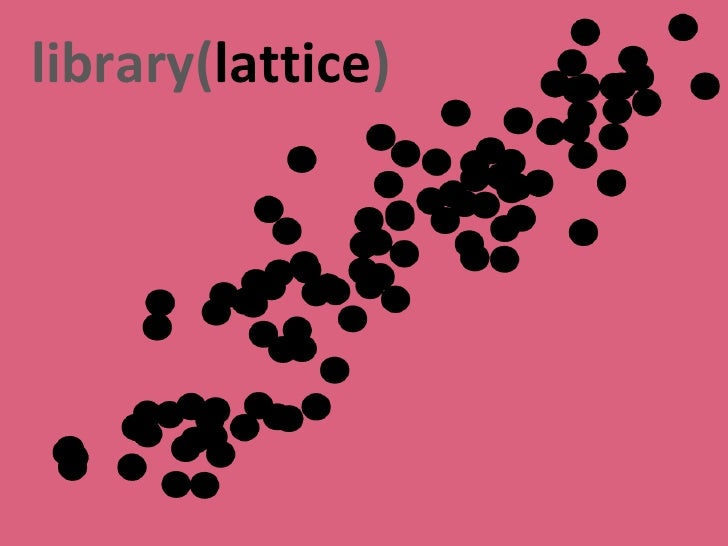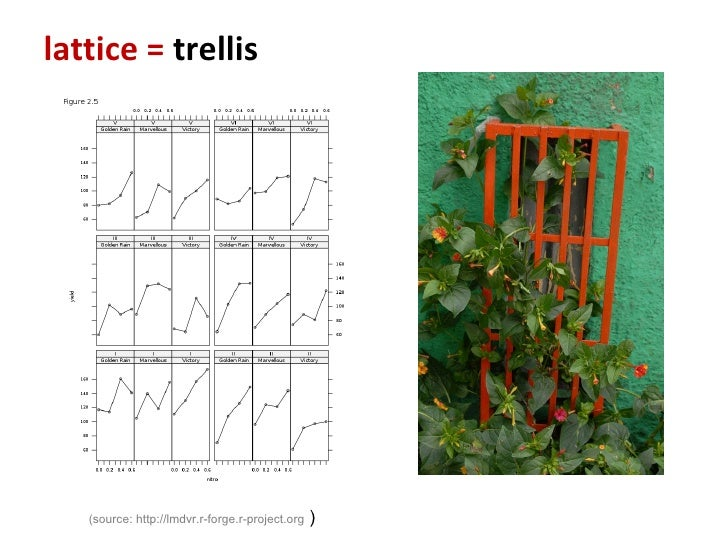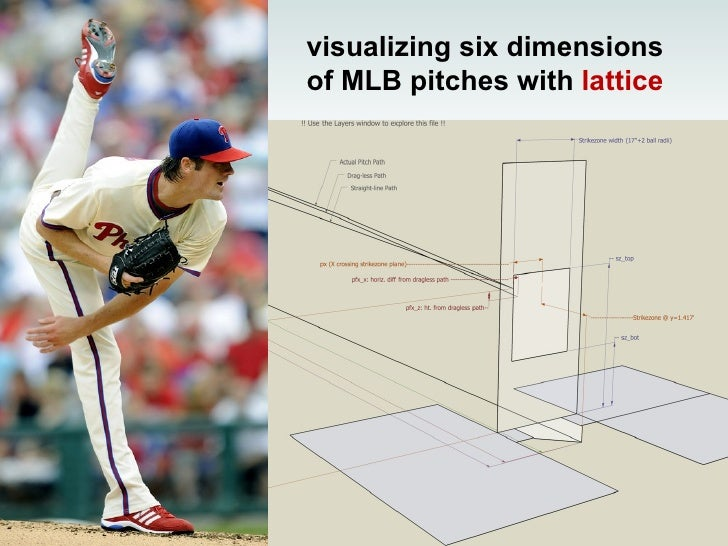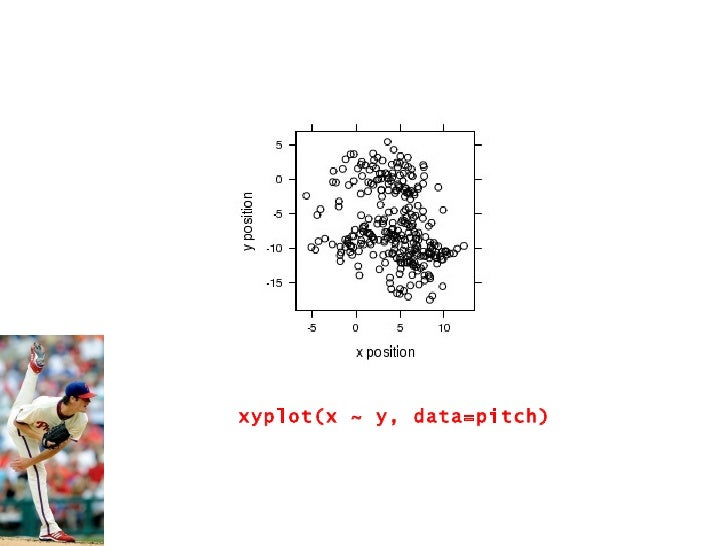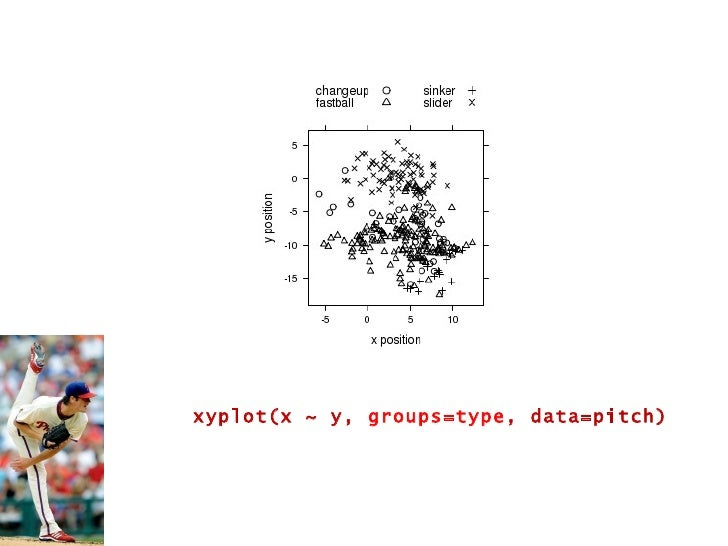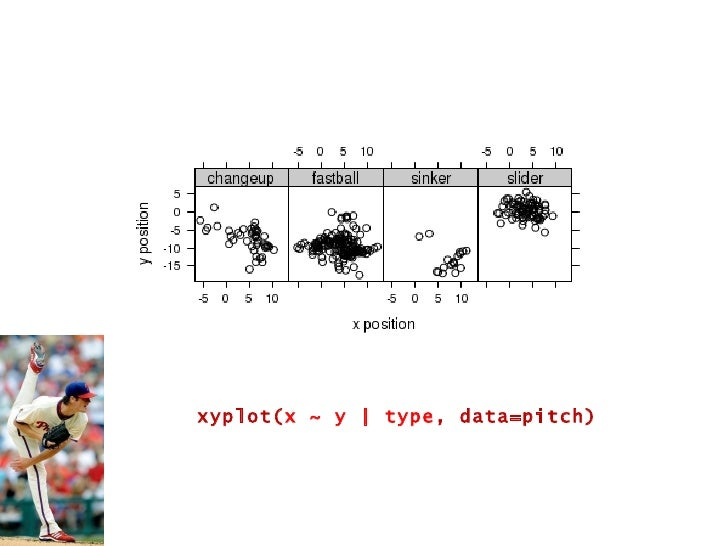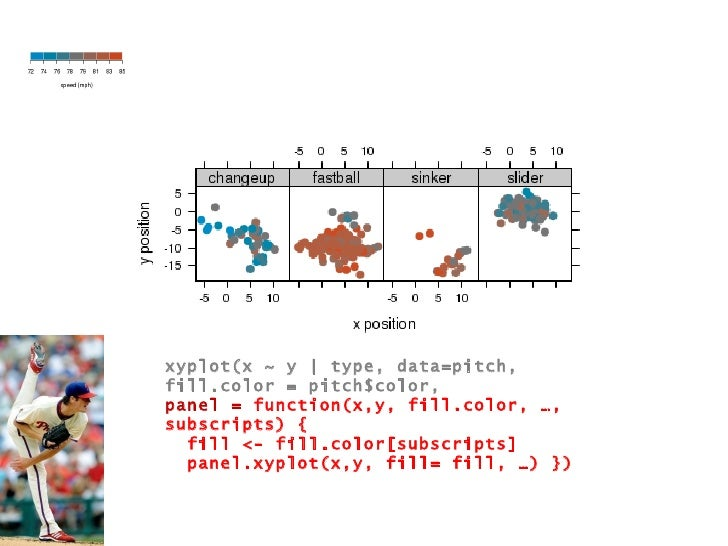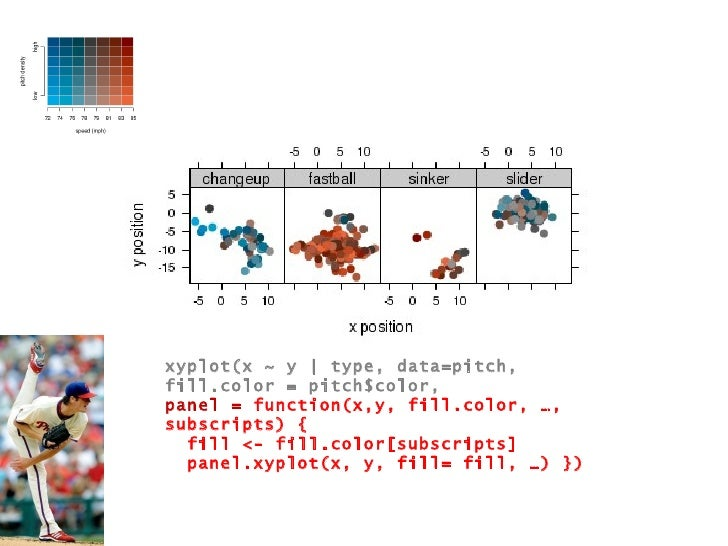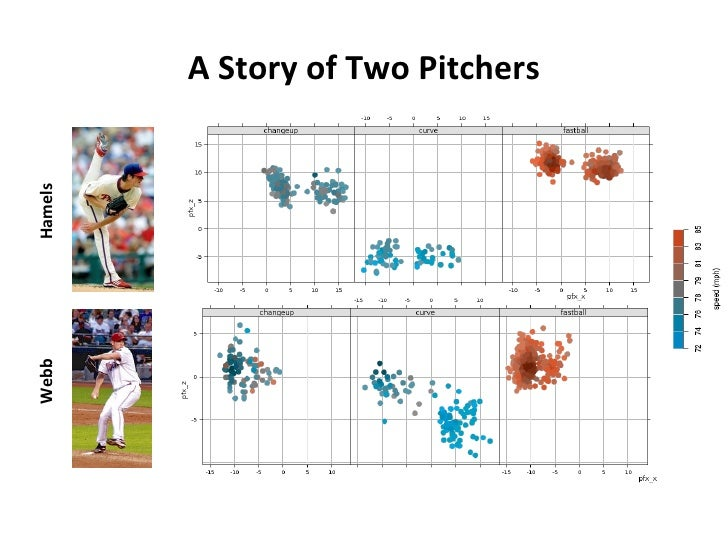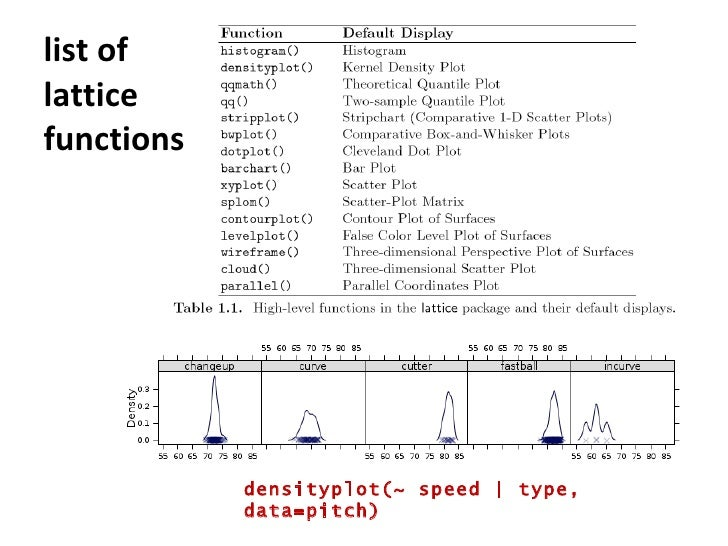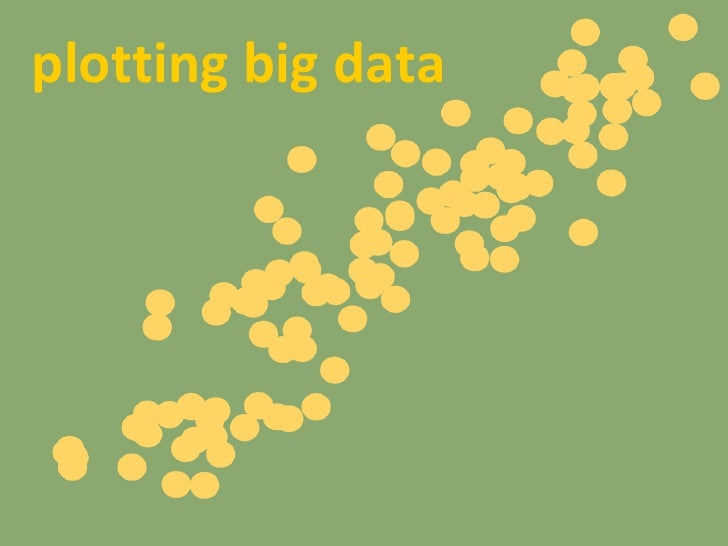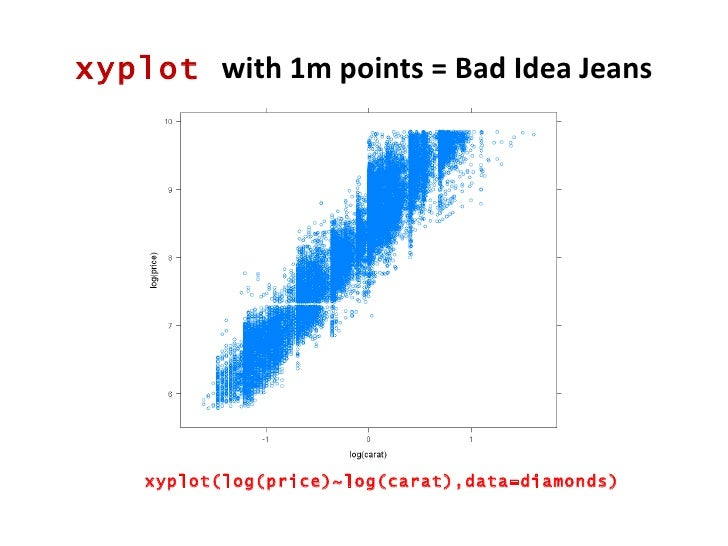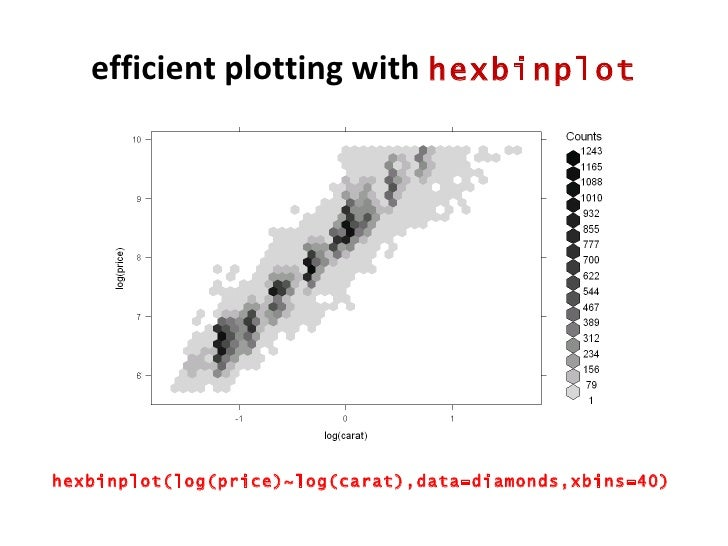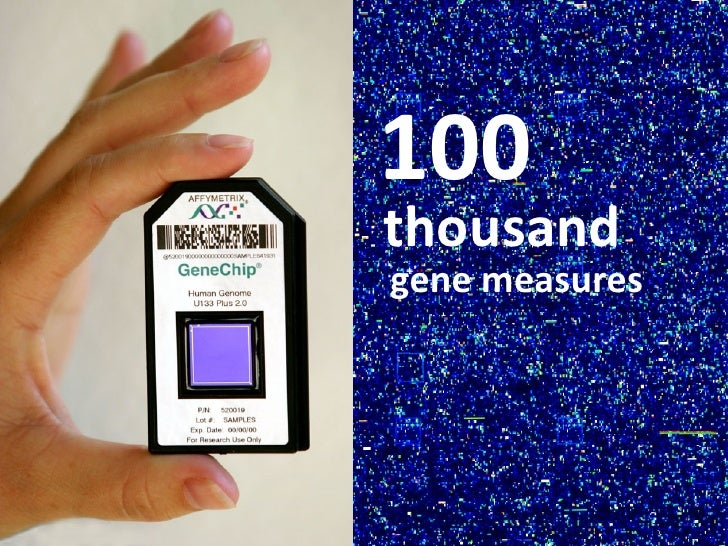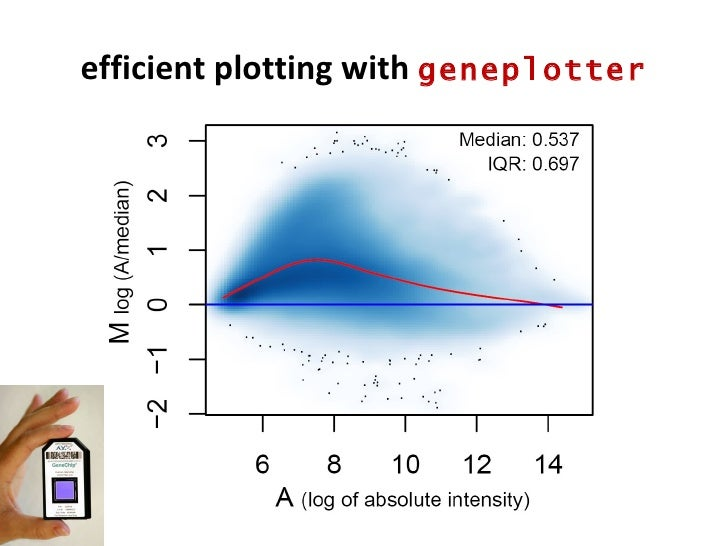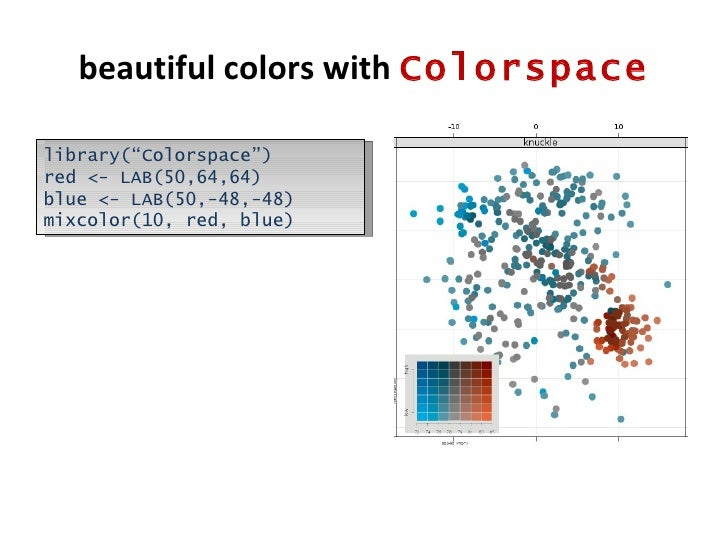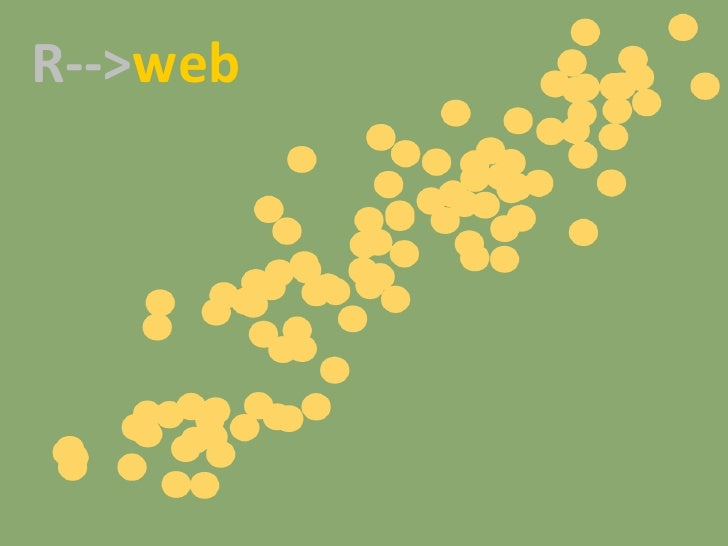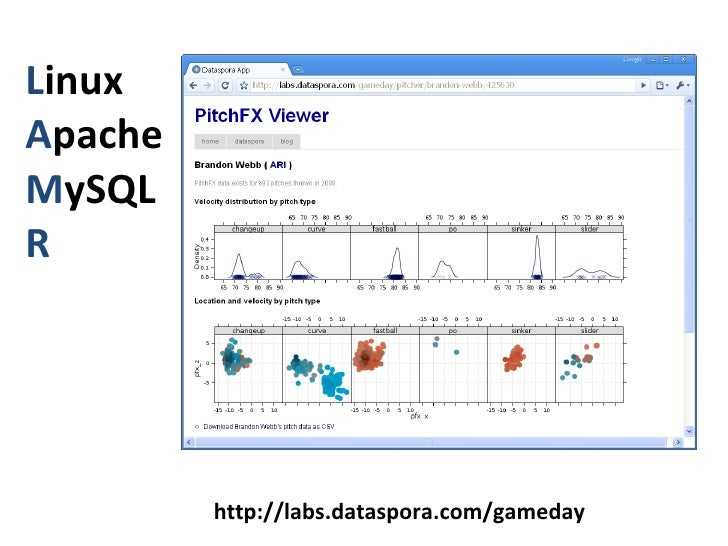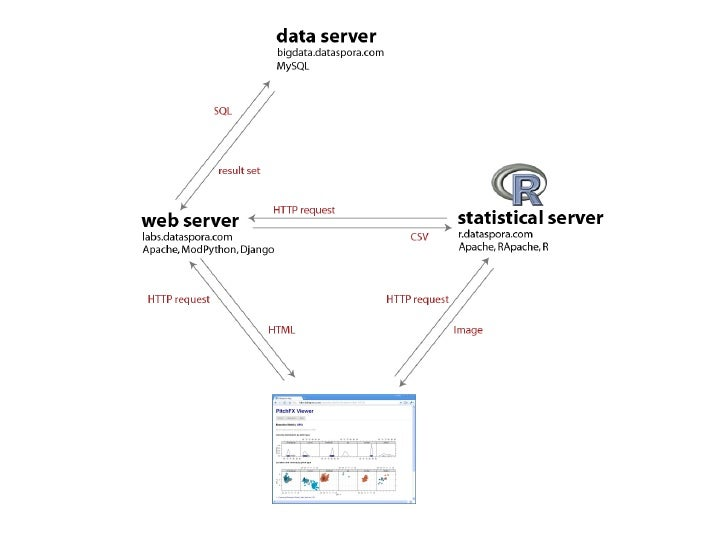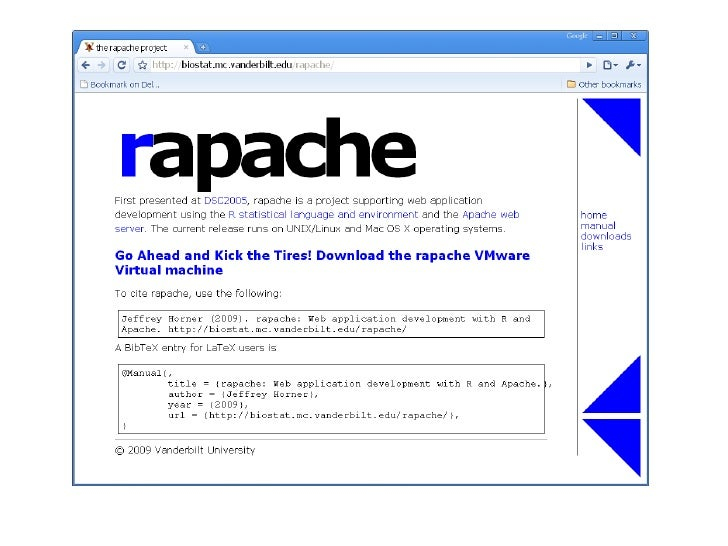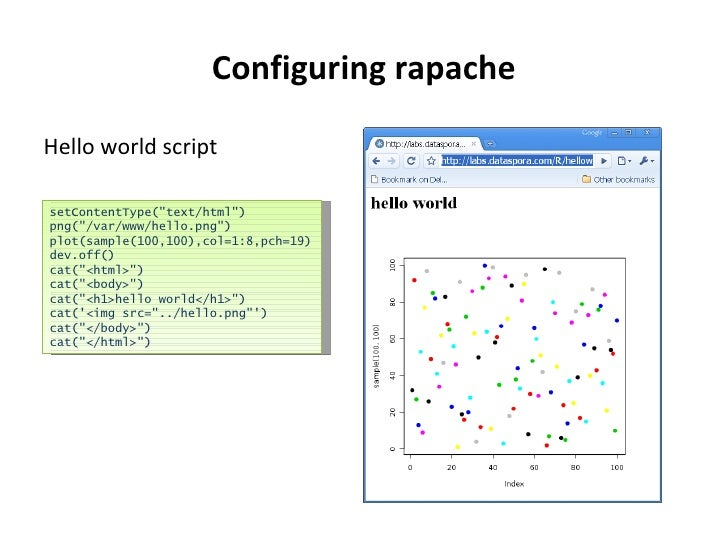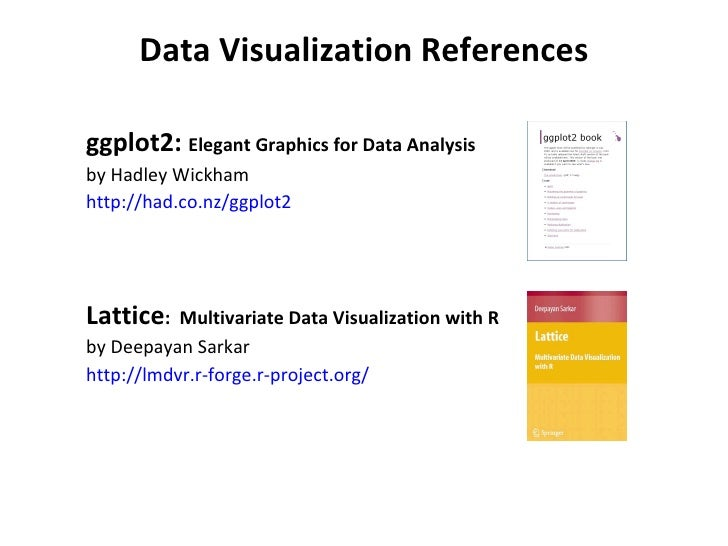Upcoming SlideShare
×
• Full Name
Comment goes here.

Are you sure you want to Yes No• Be the first to comment

### A Survey Of R Graphics

1. 1. A Survey of R Graphics June 18 2009 R Users Group of LA Michael E. Driscoll Principal, Dataspora [email_address] www.dataspora.com
2. 2. “ The sexy job in the next ten years will be statisticians…” - Hal Varian
3. 4. (from Jessica Hagy’s thisisindexed.com) Hypothesis
4. 5. gdp <- read.csv('gdp.csv') hours <- read.csv('hours.csv') gdp.hours <- merge(hours,gdp) gdp.hours\$freetime <- 4380 - gdp.hours\$hours attach(gdp.hours) plot(freetime ~ gdp) m <- lm(freetime ~ gdp,data=gdp.hours) abline(m,col=3,lw=2) pm <- loess(freetime ~ gdp) lines(spline(gdp,fitted(pm))) Munge & Model
5. 6. Visualization library(ggplot2) qplot(gdp,freetime, data=gdp.hours, geom=c(&quot;point&quot;, &quot;smooth&quot;), span=1)
6. 7. basic graphics
7. 8. R’s Two Graphics Systems
8. 9. plot() graphs objects plot(freetime ~ gdp, data=gdp.hours) model <- lm(freetime ~ gdp, data=gdp.hours) ab line(model)
9. 10. plot() graphs objects abline(model, col=&quot;red&quot;, lwd=3 )
10. 11. par sets graphical par ameters par( pch =20, cex =5, col =&quot;#5050a0 BB &quot;) RGB hex alpha blending! help(par) plot(freetime ~ gdp, data=gdp.hours)
11. 12. par sets graphical par ameters parameters for par() pch col adj srt pt.cex graphing functions points() text() xlab() legend()
12. 13. Paneling Graphics <ul><li>By setting one parameter in particular, mfrow , we can partition the graphics display to give us a m ultiple f ramework in which to panel our plots, row wise. </li></ul><ul><li>par(mfrow = c( nrow, ncol)) </li></ul>Number of rows Number of columns
13. 14. Paneling Graphics <ul><li>par(mfrow=c(2,2)) </li></ul><ul><li>hist (D\$wg, main='Histogram',xlab='Weight Gain', ylab ='Frequency', col=heat.colors(14)) </li></ul><ul><li>boxplot (wg.7a\$wg, wg.8a\$wg, wg.9a\$wg, wg.10a\$wg, wg.11a\$wg, wg.12p\$wg, main='Weight Gain', ylab='Weight Gain (lbs)', </li></ul><ul><li>xlab='Shift', names = c('7am','8am','9am','10am','11am','12pm')) </li></ul><ul><li>plot (D\$metmin,D\$wg,main='Met Minutes vs. Weight Gain', xlab='Mets (min)',ylab='Weight Gain (lbs)',pch=2) </li></ul><ul><li>plot (t1,D2\$Intel,type=&quot;l&quot;,main='Closing Stock Prices',xlab='Time',ylab='Price \$') </li></ul><ul><li>lines(t1,D2\$DELL,lty=2) </li></ul>
14. 15. Paneling Graphics
15. 16. Working with Graphics Devices <ul><li>Starting up a new graphic X11 window </li></ul><ul><ul><li>x11() </li></ul></ul><ul><li>To write graphics to a file, open a device, write to it, close. </li></ul><ul><ul><li>pdf(“mygraphic.pdf”,width=7,height=7) </li></ul></ul><ul><ul><li>plot(x) </li></ul></ul><ul><ul><li>dev.off() </li></ul></ul><ul><ul><li>In Linux, the package “Cairo “ is recommended for a device that renders high-quality vector and raster images (alpha blending!). The command would read Cairo(“mygraphic.pdf”, … </li></ul></ul><ul><ul><li>Common gotcha: under non-interactive sessions, you should explicitly invoke a print command to send a plot object to an open device. For example </li></ul></ul><ul><ul><li>print(plot(x)) </li></ul></ul>
16. 17. library( ggplot2 )
17. 18. gg plot2 = g rammar of g raphics
18. 19. gg plot2 = g rammar of g raphics
19. 20. Visualizing 50,000 Diamonds with ggplot2
20. 21. qplot (carat, price, data = diamonds)
21. 22. qplot( log (carat), log (price), data = diamonds) qplot(carat, price, log=“xy” , data = diamonds) OR
22. 23. qplot(log(carat), log(price), data = diamonds, alpha = I(1/20) )
23. 24. qplot(log(carat), log(price), data = diamonds, alpha = I(1/20), colour=color )
24. 25. Achieving small multiples with “facets” qplot(log(carat), log(price), data = diamonds, alpha=I(1/20)) + facet_grid(. ~ color)
25. 26. qplot(color, price/carat, data = diamonds, alpha = I(1/20), geom=“jitter” ) qplot(color, price/carat, data = diamonds, geom=“boxplot” ) old new
26. 28. library( lattice )
27. 29. lattice = trellis <ul><ul><li>(source: http://lmdvr.r-forge.r-project.org ) </li></ul></ul>
28. 30. visualizing six dimensions of MLB pitches with lattice
29. 31. xyplot(x ~ y, data=pitch)
30. 32. xyplot(x ~ y, groups=type , data=pitch)
31. 33. xyplot( x ~ y | type , data=pitch)
32. 34. xyplot(x ~ y | type, data=pitch, fill.color = pitch\$color, panel = function(x,y, fill.color, …, subscripts) { fill <- fill.color[subscripts] panel.xyplot(x,y, fill= fill, …) })
33. 35. xyplot(x ~ y | type, data=pitch, fill.color = pitch\$color, panel = function(x,y, fill.color, …, subscripts) { fill <- fill.color[subscripts] panel.xyplot(x, y, fill= fill, …) })
34. 36. A Story of Two Pitchers Hamels Webb
35. 37. list of lattice functions densityplot(~ speed | type, data=pitch)
36. 38. plotting big data
37. 39. xyplot with 1m points = Bad Idea Jeans xyplot(log(price)~log(carat),data=diamonds)
38. 40. efficient plotting with hexbinplot hexbinplot(log(price)~log(carat),data=diamonds,xbins=40)
39. 41. 100 thousand gene measures
40. 42. efficient plotting with geneplotter
41. 43. beautiful colors with Colorspace library(“Colorspace”) red <- LAB(50,64,64) blue <- LAB(50,-48,-48) mixcolor(10, red, blue)
42. 44. R--> web
43. 45. L inux A pache M ySQL R http://labs.dataspora.com/gameday
44. 48. Configuring rapache <ul><li>Hello world script </li></ul>setContentType(&quot;text/html&quot;) png(&quot;/var/www/hello.png&quot;) plot(sample(100,100),col=1:8,pch=19) dev.off() cat(&quot;<html>&quot;) cat(&quot;<body>&quot;) cat(&quot;<h1>hello world</h1>&quot;) cat('<img src=&quot;../hello.png&quot;') cat(&quot;</body>&quot;) cat(&quot;</html>&quot;)
45. 49. Data Visualization References <ul><ul><li>ggplot2: Elegant Graphics for Data Analysis </li></ul></ul><ul><ul><li>by Hadley Wickham </li></ul></ul><ul><ul><li>http://had.co.nz/ggplot2 </li></ul></ul><ul><ul><li>Lattice : Multivariate Data Visualization with R </li></ul></ul><ul><ul><li>by Deepayan Sarkar </li></ul></ul><ul><ul><li>http://lmdvr.r-forge.r-project.org/ </li></ul></ul>